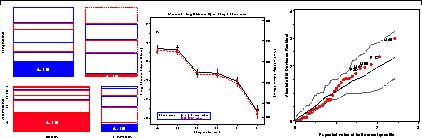# Chapter 7. Loglinear and logit modelsLoglinear models are most easily interpreted through visualizations, including mosaic displays and plots of associated logit models. As with logistic regression, diagnostic plots and influence plots help to assure that the fitted model is an adequate summary of associations among variables. 7.1. Introduction 7.2. Loglinear models for counts 7.2.1. Loglinear models as discrete ANOVA models 7.2.2. Loglinear models as discrete GLMs 7.2.3. Loglinear models for three-way tables 7.3. Fitting loglinear models 7.3.1. Goodness-of-fit tests 7.3.2. Software 7.3.3. Using PROC CATMOD 7.3.4. Using PROC GENMOD 7.3.5. Using SAS/INSIGHT 7.4. Logit models 7.4.1. Plotting results for logit models 7.4.2. Zero frequencies 7.5. Models for ordinal variables 7.5.1. Loglinear models for ordinal variables 7.5.2. Adjacent category logit models 7.5.3. Cumulative logit models 7.6. An extended example 7.6.1. A fresh look 7.7. Influence and diagnostic plots for loglinear models 7.7.1. Residuals and diagnostics for loglinear models 7.7.2. Half-normal probability plots of residuals 7.7.3. Model diagnostics with PROC GENMOD 7.7.4. Model diagnostics with PROC CATMOD 7.8. Multivariate responses 7.8.1. Examining relations 7.9. Chapter summary